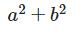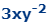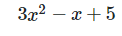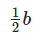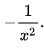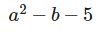## Polynomials

Polynomial by definition means many terms. A term is an expression consisting of constants, variables, and exponents. A polynomial consists of terms separated by addition/subtraction.

It is important to note that some expressions, while contains many terms, are in fact not polynomials.

Polynomials cannot contain division by a variable. Ex: $$2y^2+ \cfrac {7x}{1+x}$$ is not a polynomial as it contains division by a variable

Polynomials cannot contain negative exponents. Ex: $$2y^{-2}+7x-4$$ is not a polynomial because it is the same as division by a variable after converting $$2y^{-2} = \cfrac {2}{y^{2}}$$.

Polynomials cannot contain fractional exponents. Ex: $$3x+2y^{1/2}-1$$ is not a polynomial.

Polynomials cannot contain radicals(root). Ex: $$2y^2 + \sqrt {3x} + 4$$ is not a polynomial.

## Degree of a Polynomial

The degree of any individual term of a polynomial is determined by the exponent of its variable. For terms that contain more than one variable the degree would be the sum of the exponents on those variables. The degree of a polynomial is simply the degree of the highest term. The leading coefficient is the coefficient on the term with the highest degree.

What is the degree of the $$ab^2+a^2b^3−a^3b$$?

## Describing Polynomials

Other than degrees, a polynomial can also be categorized by terms, such that a Monomial has one term, a Binomial have two terms, and a Trinomial with three terms.

Try and fit the following polynomials into their correct description.